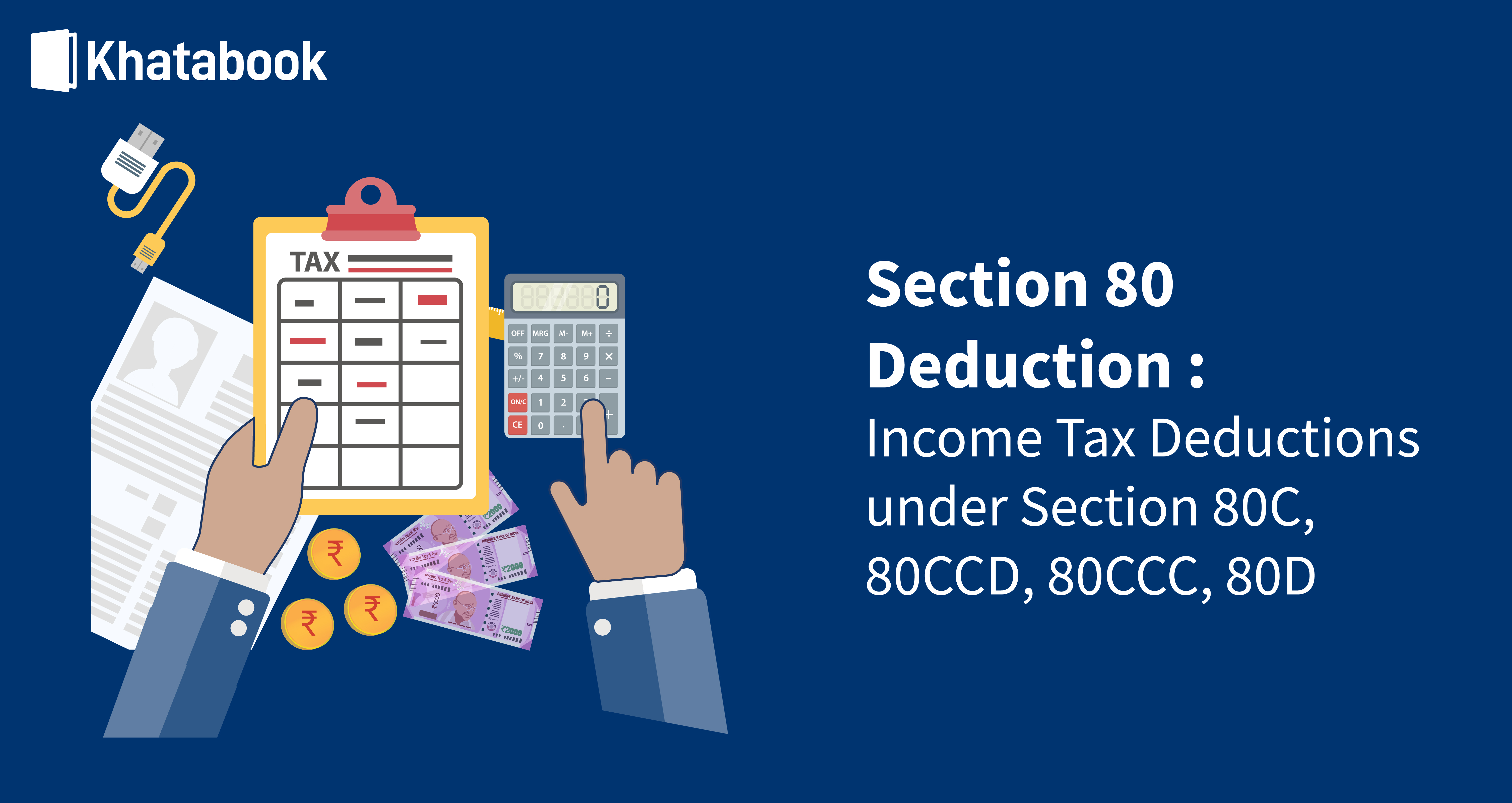# Depreciation Under Income Tax Act

Depreciation is allowed on assets that are possessed by individuals or corporates. This is calculated in whole or in part depending on the possession proportion of the asset under possession by respective individuals.

Calculating depreciation rate as per income tax is of prime importance to those who would like to know as to what is the depreciated value of the asset/s that they are holding with them. This applies to both tangible and intangible assets held individually or as part thereof. It is vital to understand depreciation basics as this forms part of the income tax applicable to them for every assessment year.

## What does Depreciation Mean?

The concept of depreciation has a lot to do with the profit and loss account of a company or the personal finances. This deals primarily with income tax depreciation rates wherein the value of an asset is effectively written off during the period of usage or the actual life of the asset. At the end of the writing, the asset's residual value remains as per income tax rules and regulations as prevalent from time to time under Indian Income Tax rules.

## Significance of depreciation rate

For residents of India, depreciation of assets is calculated based on the depreciation rate as per the income tax act. It is a well-known fact that when you buy an asset, with time, the value of the asset goes down, which implies that the value of the asset depreciates. This is true of various assets, including vehicles, buildings, electronic items for commercial and personal use.

One must remember that holding an asset itself provides enough grounds for claiming depreciation irrespective of whether the asset is being used or not. One may have 5 computers in possession but using only 4 of them. In such a case, depreciation can be claimed for all 5 of the assets. This would be as per the prescribed computer depreciation rate and not in the case only of the 4 which have been used productively.

## What are the forms of depreciation as per the Income Tax Act?

### 1. Straight Line method/Written Down Value Method

The primary form of depreciation methodologies is the Straight Line method known as the Written Down Value Method.

• The asset under consideration is written down in the straight-line method so that its value is written down in equal periods over the asset's life.
• The periods are so uniformly taken till the salvage value of the asset is reached. The straight-line method is one of the most commonly used methods of calculating the depreciation rates of an asset.

You can use this methodology to compute the depreciation rate of all types of assets and develop the salvage value that accrues to the asset at the end of the equally divided period under which the depreciation is calculated.

For understanding depreciation rates in detail, let's use an example-

Assume you buy a computer asset for Rs 100,000, and its salvage value is taken as Rs 20,000, and the period of the asset is taken as 5 years.

• In such a case, the per year depreciation on computer value would be Rs 16,000 per year.
• The computation comes to the cost of the computer less the salvage value of the computer divided by the equal periods over which the computer is depreciated, i.e. 5 years which yield a per year depreciation value of Rs 16,000.
• In this specific case, the asset's written down value (WDV) would be Rs 20,000, which is the salvage value at the end of the mentioned 5 years.
• If the asset is acquired in a particular year, the depreciation would be WDV based on the asset's actual cost.
• However, if a business acquired the asset in a previous year, the WDV would consider the actual cost of the asset and deduct the depreciation as applicable of the prior year to arrive at the current year depreciation rate.

Also Read: Section 80EE: Home Loan Tax Incentives Under the Income Tax Act 1961

### 2. Double-declining balance method

There is also another form of calculating the rate of depreciation which is commonly known as the double-declining balance method of depreciation. In this method, the asset value is seen to be declining at a faster rate initially compared to the later period of depreciation. This form of depreciation is seen to be applied in those cases where the asset depreciates faster or earlier on in the life of their usage as compared to a later stage.

An example of the double-declining balance method is:

• Consider the cost of the asset and the salvage value of the asset and the number of years in which the salvage value is decided to be arrived at. Then it is divided by the number of years into two parts, where the first part of the time is when the rate of depreciation is taken as double as that of the latter part.
• In this case, the rate of depreciation of the second half of the time period would be half of that of the first part.
• Calculate the depreciation amount in the first half period. Then subtract that from the overall depreciation amount and then divide it by the remaining half period to arrive at the depreciation rate in the second half.
• The advantage of this method is that the income tax is deferred to the later part of the period of years for which the asset is being depreciated. This is a method that some companies apply for their benefit.
• For example, a business purchased a truck for Rs 20,00,000. Assume it will last for 10 years with a salvage value of Rs 5,00,000. Under the straight line method (SLM) of depreciation, the depreciation rate would be 10% per year as the expected life is 10 years. Now double the depreciation rate per year, 20% and deduct the depreciation on the remaining value.
 Year Asset Value Depreciation @ 20% 1 20,00,000 4,00,000 2 16,00,000 3,20,000 3 12,80,000 2,56,000 4 10,24,000 2,04,800 5 8,19,200 1,63,840 6 6,55,360 1,31,072 7 5,24,288 24,288 Salvage Value 5,00,000

### 3. Units of production method

The other form of calculating depreciation is the units of a production method of depreciation as per income tax.

• This form of depreciation is used more in industrial scenarios based on the level or frequency of usage of an asset.
• In this form, if the asset is being used more at a particular point in time to produce product units, its deprecation will be more than those time frames where the asset usage is less.
• This is a function of the wear and tear of an asset due to usage rate and not based on uniform usage of the asset.
• This is also a function of the customer demand for a particular product which may vary at different points in time. For instance, if you are calculating the depreciation of a loom used in textile production, the usage of the loom will be more in the festival seasons than the lean seasons.

Hence you would calculate higher deprecation during the festive season than the lean season when the loom is idle and is not being used much.

## Block of Assets concept in Depreciation

There is a concept in depreciation in which depreciation is calculated not on a single asset but a block of assets. This consists of assets taken as one set of units to calculate depreciation.

• This concept is widely used in the manufacturing sector wherein the block of assets considered takes into account plant and machinery and associated building/s as one block.
• This is also valid for intangible assets.
• Some intangible assets that can be considered are patents and copyrights, which have a specific period of validity and value to the company that owns these intangible assets.

In the commercial sector, the Block of Assets concept in Depreciation takes franchises and licences into account. The company sells these to other associates who want to partner with the parent company for a specific period.

• In a sense, for businesses, all these assets are not taken separately but as a single block as they work in tandem or unison for a common goal that enhances the top line and bottom-line of the commercial venture in which they are used. In the example above, the building depreciation rate would not be taken by a business as a separate rather. It would be considered part of the asset block estimated for depreciation.
• One must also keep in mind that this asset block concept is rarely applicable in individual cases. If you are looking at depreciation on car as per income tax act, you cannot take as a block of assets with electronic items like a fridge or a washing machine that you are using in your house!
• Each of the items of individual users has different rates, and rules of depreciation and depreciation calculation would be considered the same according to prevalent IT rules and regulations.

### Mandatory conditions for claiming depreciation under asset block

There are certain mandatory conditions that a business must fulfil for claiming depreciation, such as:

• The assets under consideration must be owned by the person being assessed either in full or in part
• The assets under assessment must be used for the profession or business by the owner during the year. If it is used for non-business purposes, it would be necessary to assess what part of the asset usage was for business or commercial purposes. This assessment would be to calculate the depreciation to be claimed, which would be in proportion to the business usage of the assets.
• The cost of land is not to be taken as part of assets for depreciation.
• If there is more than one asset owner, the depreciation claimed would be proportionate to the ownership. For instance, if one owner owns 70% of the asset block and another 30%, the ratio of the depreciation to be claimed would be in the ratio of 70:30.
• After the financial year 2002-03, depreciation has been mandatory as per IT rules. This is independent of whether the taxpayer has claimed it under IT claims or not.

## Depreciation in case of amalgamation or demerger

There could be scenarios where companies go through a merger or an acquisition or a demerger or devolution into separate companies.

• In such cases, they would be proportionately divided in terms of the applicable depreciation as per the terms of amalgamation or demerger.
• In the case of amalgamation, the depreciation would accrue to the amalgamated entity post amalgamation.
• In the case of devolution, conversely, the demerged or devolved units would be able to claim depreciation proportionate to their demerger conditions.

​​​​​​​Also Read: Deductions under Section 80: Section 80C, 80CCC, 80CCD & 80D Income Tax

## FAQs

1. What is the depreciation on a car as per income tax act?

The car Depreciation rate for cars that are not used as commercial vehicles for rent the depreciation rate is 15%. It is 30% for cars that are bought between 23rd August 2019 and before 1st April 2020 and put into use before 1st April 2020

2. What is the AC depreciation rate?

The rate of depreciation for air conditioners is 40%.

3. What is the depreciation rate on computers?

The depreciation of computers, as well as computer software, is prescribed at 40%.

4. What is the building depreciation rate

There are different rates of depreciation as applicable on different types of buildings as per their usage as below:

• For purely residential buildings that are not used for lodging and boarding, the depreciation rate is 5%
• For hotels and boarding lodges, the depreciation rate is 10%
• Temporary structures or constructions used for dwelling attract a depreciation rate of as high as 40%.

5. What is depreciation on asset sold during the year as per income tax act?

This is dependent on whether the asset has been put to use in the year in which it was purchased. Even if some trial runs are conducted on the asset, it will be deemed eligible to attract depreciation. If the asset is used for less than 180 days in the year, 50% of the normal depreciation rate is deemed the applicable depreciation rate. If an asset is put into use before the 3rd of October or 4th October if it’s a leap year, the asset can be taken as 100% of the depreciation rates

## Related Posts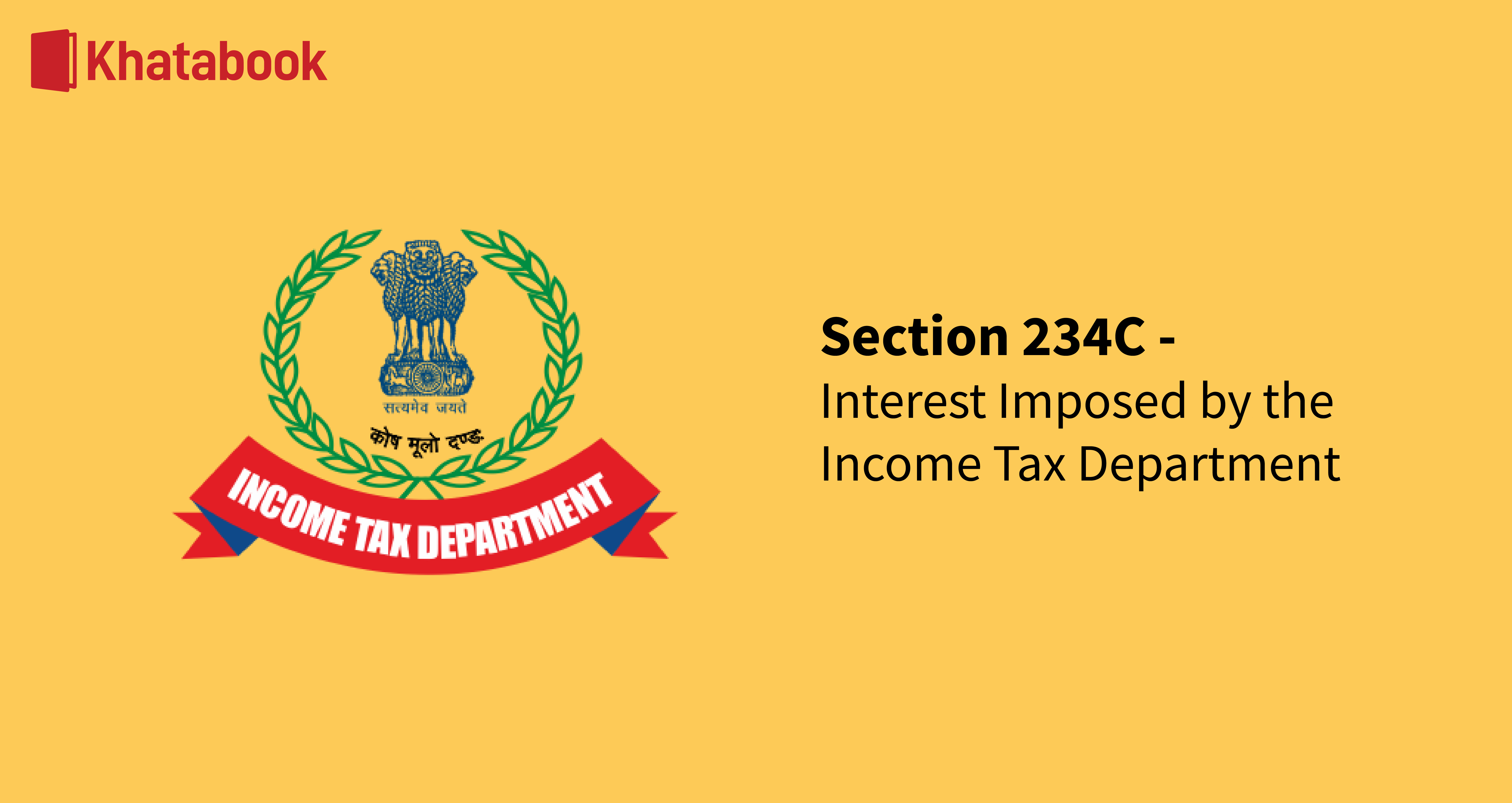### Interest Imposed by the Income Tax Department Under Section 234C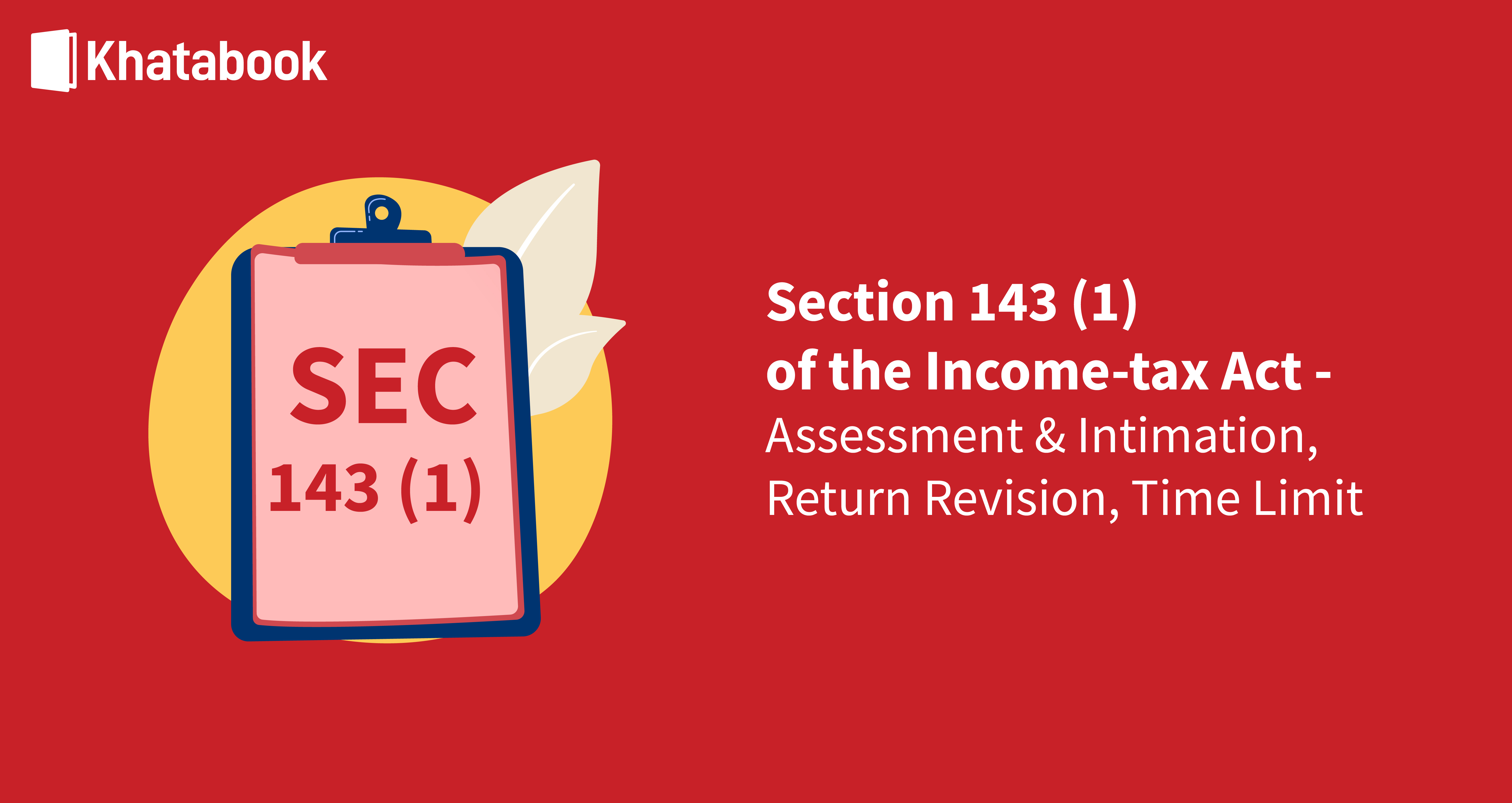### All You Need To Know About Section 143(1) of Income Tax Act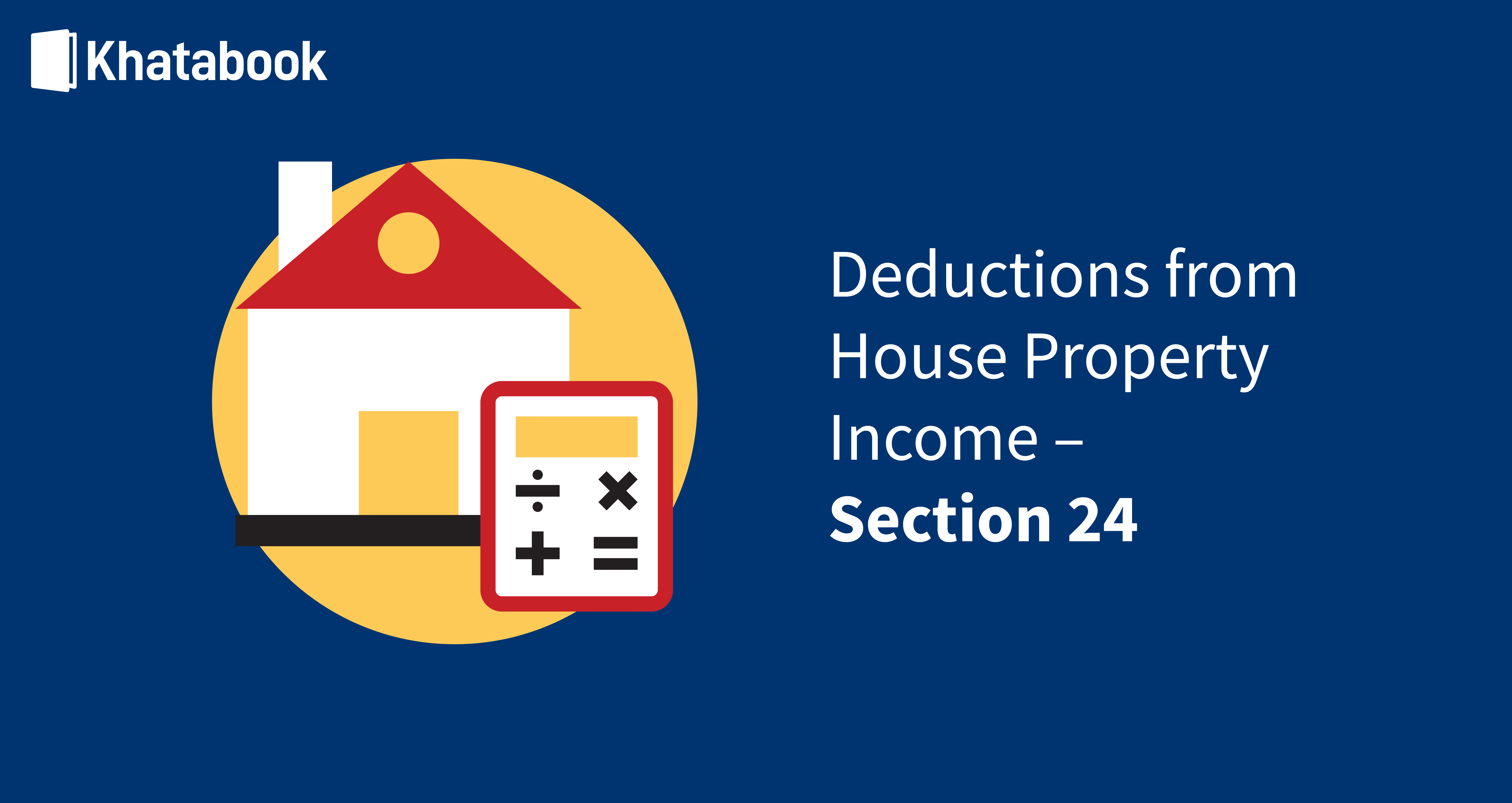### Deductions from House Property Income– Section 24### Know About Paying Taxation of Income Earned From Selling Shares### How to Get Income Certificate Online – Procedure & Format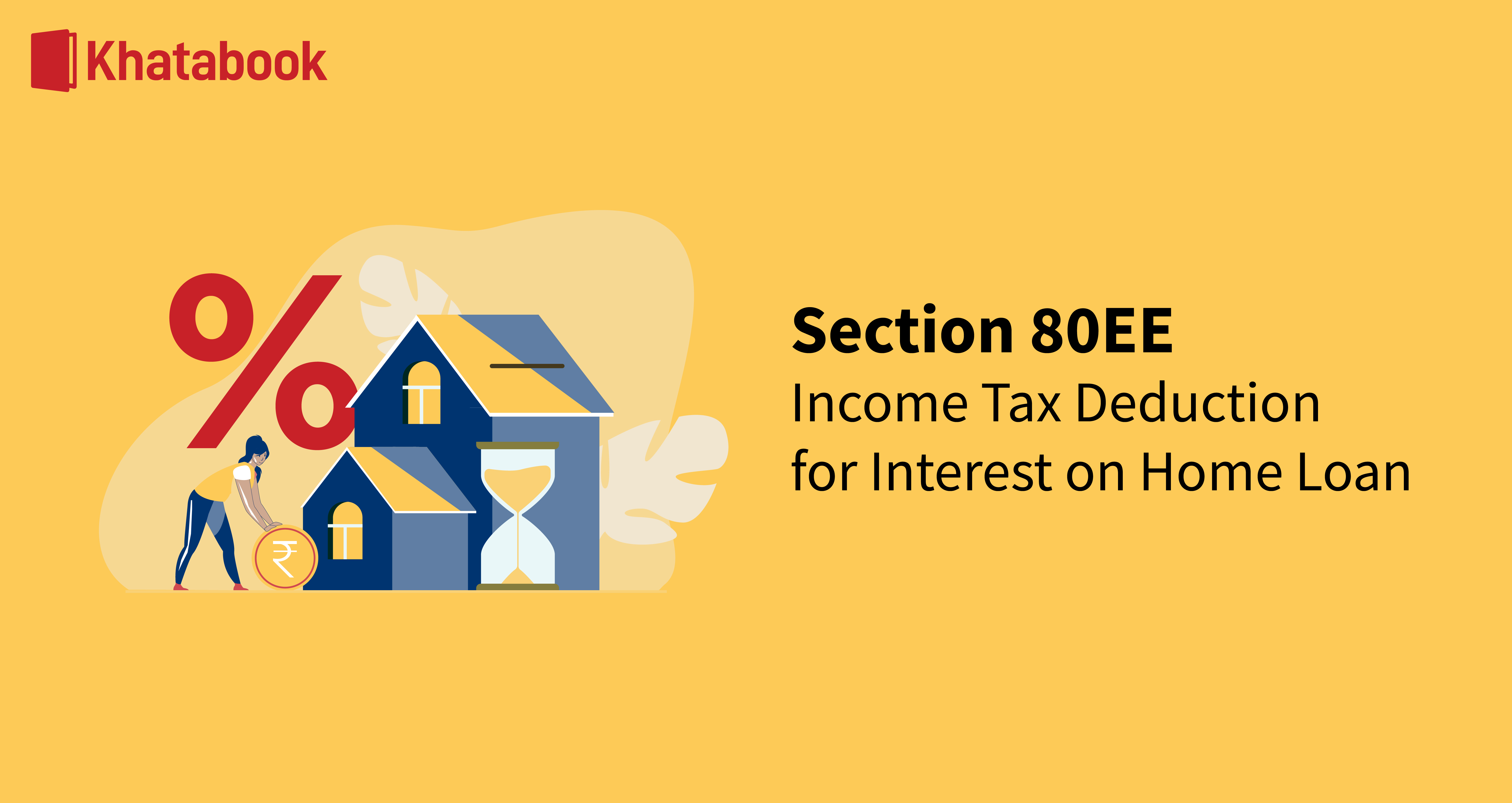### Section 80EE: Home Loan Tax Incentives Under the Income Tax Act 1961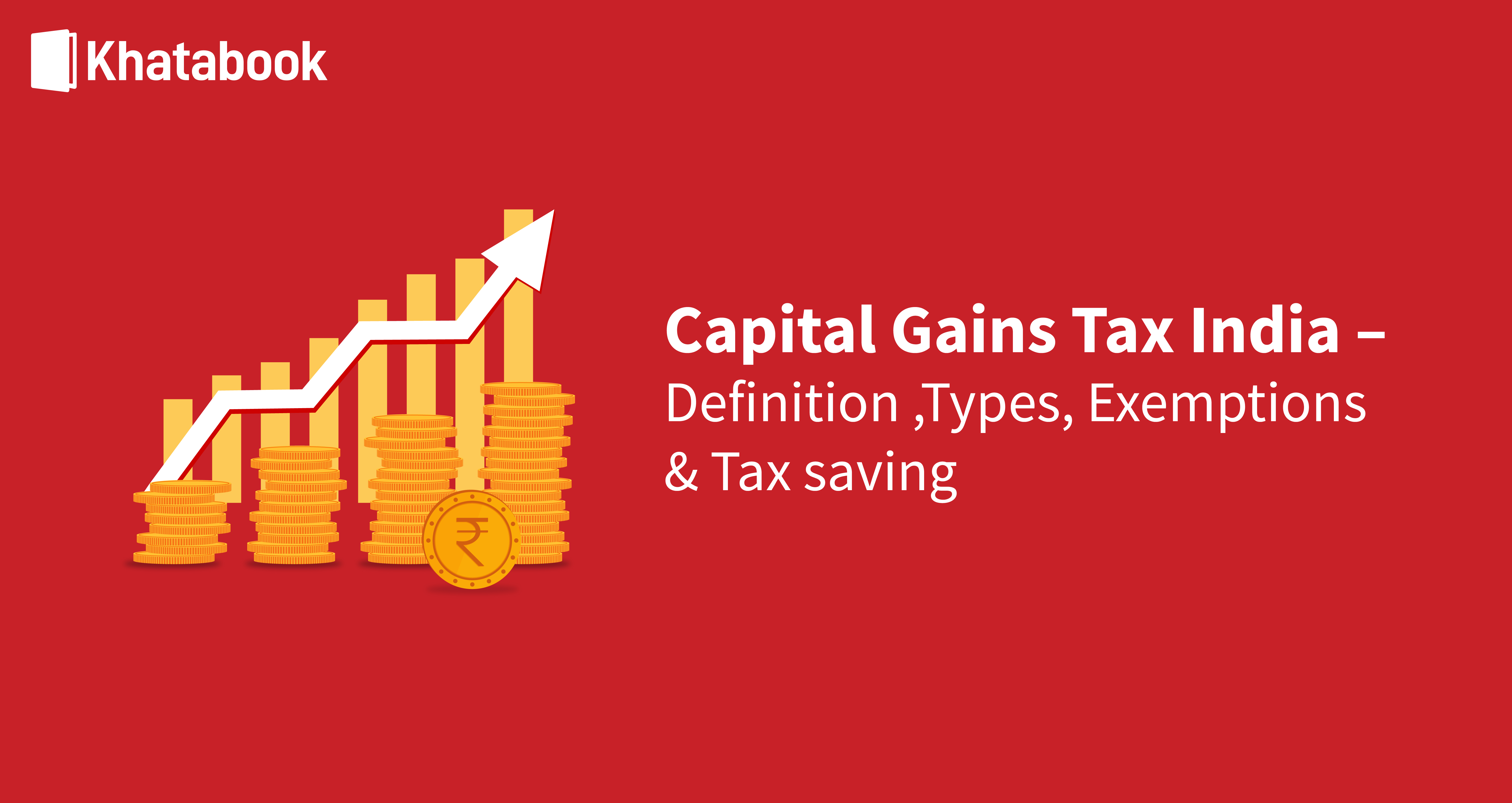### Capital Gains Tax India– Definition, Types, Exemptions & Tax Saving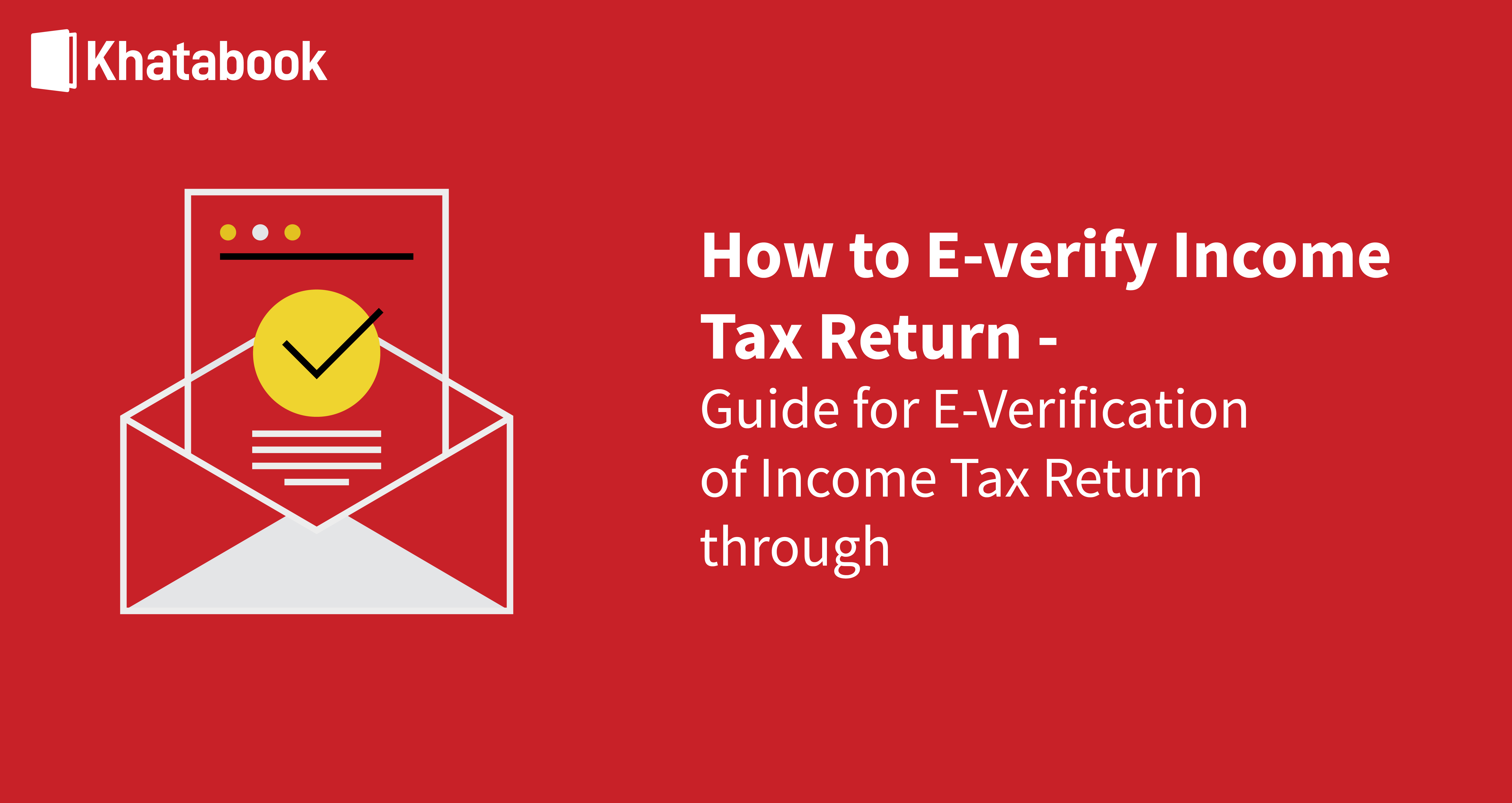### Step-by-Step Guide for E-Verifying Your Income Tax Return